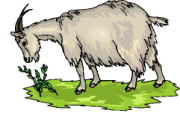# Goat Grazing around a Silo

A goat is tethered to a round silo of radius R by a rope L in length. How much area can he graze. An earlier post by Phil Todd show the answer to the question when the rope is half the circumference or shorter. He goes on to ask: "what happens when the rope is longer"? Here is the answer. Use the slider from 0 to .378 to find out.

This document requires an HTML5-compliant browser.
 L (length of rope) r (radius of silo) tether position 0 0 0.379 z

To find the goat's grazing area when the rope is longer than πR, we must stop the rotation when the tip of the rope reaches the center line (pretend it is a fence) and calculate the yellow area by integration. Then add the triangle area and subtract the sector of the silo within the triangle. This result is doubled because the goat comes from both sides. Then the free half-circle is added for the total grazing area. The gray is the overlap. Go to http://www.tomlaidlaw.com/goatandsilo
for a complete discussion of this and other tethering problems.

App generated by Geometry Expressions